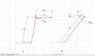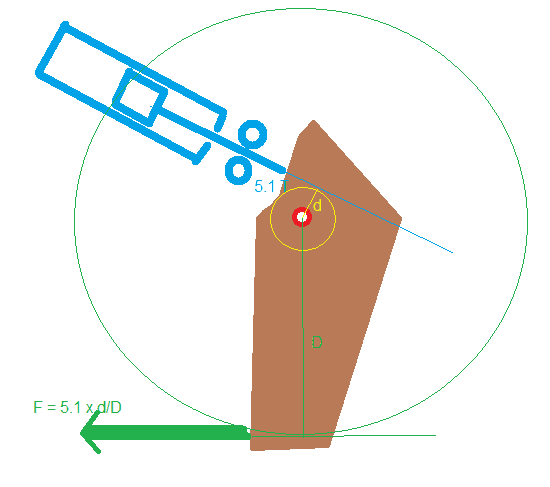# Leverage problem simplified

• Ted Farkas
In summary, Ted's problem is that he does not understand the diagrams he is looking at and does not know how to calculate the resultant force from the input and output force vectors.f

#### Ted Farkas

Hi

This is a simplified force diagram of a problem I have of some machinery. (shown in same thread)

I am trying to get the output force a

My calcs gave me 1.5 ton for A and 612 kg for B diagram.

when the leverage is not a straight seesaw - do you take arm length from pivot or is one arm taken as perpendicular height.

Also the force is a ram, do you need to workout a resultant force with the x and y-axis or can you just assume 5.1 ton is acting on the pivot arm.

I hope this makes sense.

I am working through my old engineering text but everything is a nice easy 'see saw' diagram

thanks
Ted

#### Attachments

•calc a.JPG
28.3 KB · Views: 310
I can't fathom out what your diagrams are showing. I think the witness lines may be confusing me. The lever arms seem to change in length and relative orientation?
Anyhow, I think the notion you need is, that for a rigid lever of any shape, the torques are equal to the force times the perpendicular distance from the fulcrum to the line of action of the force.

•CWatters
when the leverage is not a straight seesaw - do you take arm length from pivot or is one arm taken as perpendicular height.

Neither.

Draw extended lines representing the direction of the input and output force vectors. Then with a pair of compasses draw two circles that both have their centre on the pivot and which just touch the force vector. (Eg the extended force vectors are tangential to the circles). The two radii of these circles are the lengths of the lever arms.

I would make a diagram but it's too difficult on this cheap tablet I'm using. Will try to do one tomorrow when I'm on my pc unless someone beats me to it.

I think this is something like what CWatters had in mind.#### Attachments

•JBA
I think the above is a great method graphically; and, as long as the drawing is accurate and created using CAD, can be just precise as a mathematical solution.

Another method, rather than calculating effective lever lengths, is to determine the 5.1 ton force vector perpendicular to the upper arm and then multiply that result times the arm length to get the resulting moment of that arm at the the fulcrum point.

For the A schematic:
1. The amount of rotating force on the top arm from the 5.1 ton force = 5.1 X sin (the angle between the line of force and the top arm).
2. The resultant force perpendicular to the lower arm at its tip = the above force x 518 / 823.
3. The final horizontal force at the lever tip = the above perpendicular force x cos (the angle between a line perpendicular to the lower arm at its tip and the horizontal line, i.e, angle A).

Obviously these three steps can be reduced to a single equation.

The same procedure can be applied to the B Schematic

My result using the moment and lever method on F1 is 756 kg, so we essentially agree, based upon the reality that some of my dimensions and angles are obtained by marking and scaling of prints of your drawing.

I notice you used kg rather than Newtons for force, is due to your location or what; because I get flack about giving my force results in kg (which are actually converted units at the end of my US standard lbf and inches calculations) instead of Newtons for force from some of the SI unit posters.

My result using the moment and lever method on F1 is 756 kg, so we essentially agree, based upon the reality that some of my dimensions and angles are obtained by marking and scaling of prints of your drawing.

I notice you used kg rather than Newtons for force, is due to your location or what; because I get flack about giving my force results in kg (which are actually converted units at the end of my US standard lbf and inches calculations) instead of Newtons for force from some of the SI unit posters.

Hi,

Thanks for your help, I'm in Australia and working with agricultural equipment and farmers. I'm just using KG's because that is all the layman wants to hear or understand as it will be discussed in those terms as opposed to ;classroom', rough and ready probably.

thanks'
Ted

I think the above is a great method graphically; and, as long as the drawing is accurate and created using CAD, can be just precise as a mathematical solution.

Another method, rather than calculating effective lever lengths, is to determine the 5.1 ton force vector perpendicular to the upper arm and then multiply that result times the arm length to get the resulting moment of that arm at the the fulcrum point.

For the A schematic:
1. The amount of rotating force on the top arm from the 5.1 ton force = 5.1 X sin (the angle between the line of force and the top arm).
2. The resultant force perpendicular to the lower arm at its tip = the above force x 518 / 823.
3. The final horizontal force at the lever tip = the above perpendicular force x cos (the angle between a line perpendicular to the lower arm at its tip and the horizontal line, i.e, angle A).

Obviously these three steps can be reduced to a single equation.

The same procedure can be applied to the B Schematic

I agree, better to calculate it rather than do it graphically.

Drawing circles just helps you recognise where the right angle triangle is you are working with for the trig.